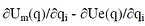top of page

# Extracting force parameter from distributed (Pressure) load

In the process of design optimization, a MEMS designer performs multiple simulations using Finite Element (FEM) based simulations. While FEM simulation is reliable in IntelliSuite software, we also recommend the users to perform, Reduced Ordered Modeling (ROM) or System Model Extraction (SME) which plays a vital role in benchmarking the FEM model using system-level simulation (Lagrangian based, the outcome of SME simulation also aids in Integration with other EDA tools). Most of the simulation settings are straight forward in FEM using the GUI, In the case of system-level simulation through the SME, input parameter such as distributed load is considered with an equivalent force. Hence, it is important to extract the parameter from distributed load. We demonstrate how a distributed load (Pressure) can be converted to equivalent force for simulating it at the system level.

The electro-mechanical system model equation can be summarised as1

The system model allows the user to apply arbitrary force loading to any number of points on the structure. While this is very useful for applications such as micromirrors and inertial devices. Devices such as pressure sensors have distributed loads applied across the membrane. Hence, we need a methodology to model the distributed pressure load by an equivalent force.

Consider a representative node on the structure with one applied force. The electro-mechanical equation can be written as:2

We need to find an equivalent force Fj that can be used to simulate the applied pressure P. For most pressure sensors, operating in the linear range, the displacement vs force can be represented as a linear spring given by the equation:3

Where the term in Left Hand Side is the difference of the applied force and "del qi" is calculated "scaling factor for mode i" from the software during the system-level simulation using the applied two different forces. kf (the spring constant) is the linear term coefficient, the term,                                      which can be calculated from the above equation.For the pressure sensor, typically the primary mode dominates the device performance. Typically all of the energy is contained within the first mode. Therefore, the above macro model can be used to describe the dynamic behavior of the sensor. More specifically, the equation4

Can be written as5

Where the term in the left-hand side is the primary mode shape function, qi(t) represents the coefficients for the primary mode i (which is also referred as "scaling factor for mode i")

Using TEM (thermal-electromechanical module) module, with the same boundary conditions that were used to derive the macromodel, the user can derive the pressure-displacement curve. In the linear range, this can be approximated by:6

where kp is the spring constant for a distributed pressure loading (it can be calculated from the slope of the pressure vs displacement curve) it is given as calculated from the difference of displacement Δ (ψI* qi) divided by the pressure difference ΔP.

Considering both system-level simulation ( performed in SYNPLE module of IntelliSuite) and finite level simulation in (performed in TEM module of IntelliSuite) should have the same displacement result with the equivalent force (pressure), we have7

Therefore, we can add a conversion element to translate the pressure to force with the following expression8

If the representative node is chosen as the node with the maximum displacement, we can set ψI = 1. This leaves us with the equation9

Where kf is the slope of the force-displacement curve and kp is the slope of the pressure-displacement curve.

• The slope of the pressure Vs Displacement can be extracted by applying the pressure on the diaphragm and extracting the displacement of a node with maximum displacement. With two points (pressure & Displacement) on the slope, we can compute Kp. Similarly for Kf by applying force load.

• The value for the mode shape ψI is 1 since the selected node (for Kp and Kf) has the maximum displacement.

• By equation (9) to compute the force to be applied to the selected node for analysis in system-level simulation on SYNPLE, it is important to pick the same node (used for computing Kp and Kf) as a representative node while extracting the macromodel.

bottom of page